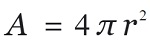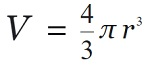# C program to calculate the surface area, volume of the Sphere

Here, we are going to learn how to calculate the surface area, volume of the Sphere using C program?
Submitted by Nidhi, on August 10, 2021

Problem Solution:

Read the radius of Sphere from the user, and then calculate the surface area and volume of the Sphere.

Surface area of the Sphere:

The formula to calculate the surface area of the Sphere is:Volume of the Sphere:

The formula to calculate the volume of the Sphere is:Program:

The source code to calculate the surface area, volume of the Sphere is given below. The given program is compiled and executed using GCC compile on UBUNTU 18.04 OS successfully.

```// C program to calculate the surface Area and
// volume of Sphere

#include <stdio.h>
#include <math.h>

int main()
{

float volume = 0;
float area = 0;

volume = (4.0 / 3) * (3.14) * pow(radius, 3);
area = 4 * (3.14) * pow(radius, 2);

printf("Volume of Sphere 	  : %f\n", volume);
printf("Surface area of Sphere: %f\n", area);

return 0;
}
```

Output:

```RUN 1:
Volume of Sphere          : 61.569649
Surface area of Sphere: 75.391403

RUN 2:
Volume of Sphere          : 4.186666
Surface area of Sphere: 12.560000

RUN 3:
Volume of Sphere          : 5572453.500000
Surface area of Sphere: 151976.000000
```

Explanation:

Here, we read the value of radius from the user. Then we calculated the surface area and volume of the Sphere using standard formulas.